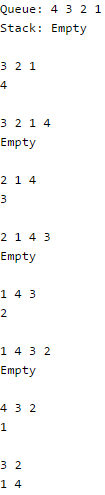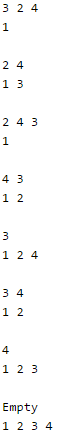Courses

# Queues MCQ - 1

## 15 Questions MCQ Test RRB JE for Computer Science Engineering | Queues MCQ - 1

Description
This mock test of Queues MCQ - 1 for Computer Science Engineering (CSE) helps you for every Computer Science Engineering (CSE) entrance exam. This contains 15 Multiple Choice Questions for Computer Science Engineering (CSE) Queues MCQ - 1 (mcq) to study with solutions a complete question bank. The solved questions answers in this Queues MCQ - 1 quiz give you a good mix of easy questions and tough questions. Computer Science Engineering (CSE) students definitely take this Queues MCQ - 1 exercise for a better result in the exam. You can find other Queues MCQ - 1 extra questions, long questions & short questions for Computer Science Engineering (CSE) on EduRev as well by searching above.
QUESTION: 1

### Following is C like pseudo code of a function that takes a Queue as an argument, and uses a stack S to do processing. void fun(Queue *Q)  {      Stack S; // Say it creates an empty stack S      // Run while Q is not empty      while (!isEmpty(Q))      {          // deQueue an item from Q and push the dequeued item to S          push(&S, deQueue(Q));      }      // Run while Stack S is not empty      while (!isEmpty(&S))      {      // Pop an item from S and enqueue the poppped item to Q      enQueue(Q, pop(&S));      }  }  Q. What does the above function do in general?

Solution:

The function takes a queue Q as an argument. It dequeues all items of Q and pushes them to a stack S. Then pops all items of S and enqueues the items back to Q. Since stack is LIFO order, all items of queue are reversed.

QUESTION: 2

Solution:
QUESTION: 3

### How many stacks are needed to implement a queue. Consider the situation where no other data structure like arrays, linked list is available to you.

Solution:

A queue can be implemented using two stacks. See following for implementation.

QUESTION: 4

How many queues are needed to implement a stack. Consider the situation where no other data structure like arrays, linked list is available to you.

Solution:

A stack can be implemented using two queues. Please see following for details.

QUESTION: 5

A priority queue can efficiently implemented using which of the following data structures? Assume that the number of insert and peek (operation to see the current highest priority item) and extraction (remove the highest priority item) operations are almost same.

Solution:
QUESTION: 6

Which of the following is true about linked list implementation of queue?

Solution:

To keep the First IFirst Out order, a queue can be implemented using linked list in any of the given two ways.

QUESTION: 7

Suppose a circular queue of capacity (n – 1) elements is implemented with an array of n elements. Assume that the insertion and deletion operation are carried out using REAR and FRONT as array index variables, respectively. Initially, REAR = FRONT = 0. The conditions to detect queue full and queue empty are

Solution:

Suppose we start filling the queue.

Let the maxQueueSize ( Capacity of the Queue) is 4.
So the size of the array which is used to implement this circular queue is 5, which is n.

In the begining when the queue is empty, FRONT and REAR point to 0 index in the array.

REAR represents insertion at the REAR index.
FRONT represents deletion from the FRONT index.

enqueue("a"); REAR = (REAR+1)%5; ( FRONT = 0, REAR = 1)
enqueue("b"); REAR = (REAR+1)%5; ( FRONT = 0, REAR = 2)
enqueue("c"); REAR = (REAR+1)%5; ( FRONT = 0, REAR = 3)
enqueue("d"); REAR = (REAR+1)%5; ( FRONT = 0, REAR = 4)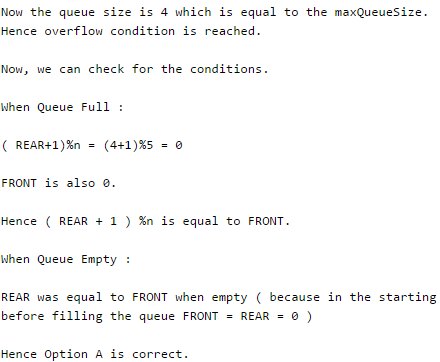QUESTION: 8

A Priority-Queue is implemented as a Max-Heap. Initially, it has 5 elements. The level-order traversal of the heap is given below: 10, 8, 5, 3, 2 Two new elements ”1‘ and ”7‘ are inserted in the heap in that order. The level-order traversal of the heap after the insertion of the elements is:

Solution:
QUESTION: 9

An implementation of a queue Q, using two stacks S1 and S2, is given below: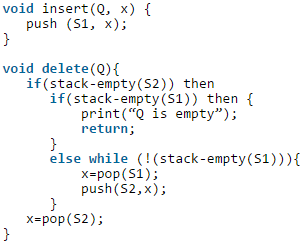Q. Let n insert and m (<=n) delete operations be performed in an arbitrary order on an empty queue Q. Let x and y be the number of push and pop operations performed respectively in the process. Which one of the following is true for all m and n?

Solution:

The order in which insert and delete operations are performed matters here. The best case: Insert and delete operations are performed alternatively. In every delete operation, 2 pop and 1 push operations are performed. So, total m+ n push (n push for insert() and m push for delete()) operations and 2m pop operations are performed. The worst case: First n elements are inserted and then m elements are deleted. In first delete operation, n + 1 pop operations and n push operation are performed. Other than first, in all delete operations, 1 pop operation is performed. So, total m + n pop operations and 2n push operations are performed (n push for insert() and n push for delete())

QUESTION: 10

The following C program is executed on a unix/Linux is: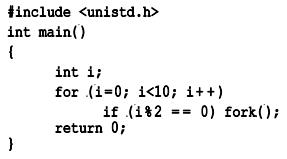The total number of child processes created is ______

Solution:
QUESTION: 11

Consider the following pseudo code. Assume that IntQueue is an integer queue. What does the function fun do?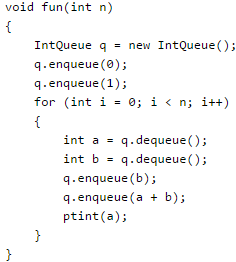Solution:

The function prints first n Fibonacci Numbers. Note that 0 and 1 are initially there in q. In every iteration of loop sum of the two queue items is enqueued and the front item is dequeued.

QUESTION: 12

Consider the following operation along with Enqueue and Dequeue operations on queues, where k is a global parameter.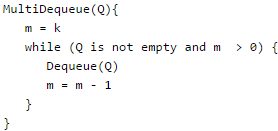Q. What is the worst case time complexity of a sequence of n MultiDequeue() operations on an initially empty queue?

Solution:
QUESTION: 13

Suppose implementation supports an instruction REVERSE, which reverses the order of elements on the stack, in addition to the PUSH and POP instructions. Which one of the following statements is TRUE with respect to this modified stack?

Solution:

To DEQUEUE an item, simply POP. To ENQUEUE an item, we can do following 3 operations 1) REVERSE 2) PUSH 3) REVERSE

QUESTION: 14

A queue is implemented using an array such that ENQUEUE and DEQUEUE operations are performed efficiently. Which one of the following statements is CORRECT (n refers to the number of items in the queue)?

Solution:

We can use circular array to implement both in O(1) time. See below article for details.

• Queue Introduction and Array Implementation
QUESTION: 15

Let Q denote a queue containing sixteen numbers and S be an empty stack. Head(Q) returns the element at the head of the queue Q without removing it from Q. Similarly Top(S) returns the element at the top of S without removing it from S. Consider the algorithm given below.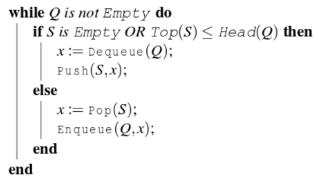Q. The maximum possible number of iterations of the while loop in the algorithm is______
[This Question was originally a Fill-in-the-Blanks question]

Solution:

The worst case happens when the queue is sorted in decreasing order. In worst case, loop runs n*n times.